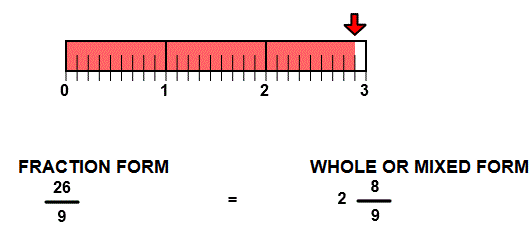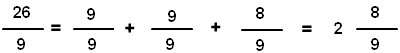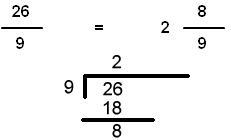# Mixed fractions with lines

## Number line models picture mixed numbers with whole number, numerator, and denominator.

WHOLE or MIXED NUMBERS
CORRECT:
ATTEMPTS:
SCORE:
PERCENT
WHOLE
NUMERATOR
DENOMINATOR
FRACTION FORM
WHOLE OR MIXED FORM

# MIXED FRACTIONS WITH LINES INSTRUCTIONS

Follow the directions in the dialog box after pressing the <START> button. The <EXPLAIN> button may be pressed to see how to do the example.

Identify Mixed Numbers uses number line models to demonstrate the meaning of whole number, numerator and denominator.

The illustration below was made by Identify Mixed Numbers Designer.

The number line model shows the fraction 269.  You are to write the fraction in mixed number form with a whole number, numerator, and denominator.

The whole numbers in the illustration are 0, 1, 2, and 3. Because the arrow is to the right of 2, you will enter 2 for the whole number. The numerator is 8 because the arrow points to the 8th of the 9 parts between 2 and 3. The denominator is 9 because there are 9 parts between 2 and 3.Other methods can be used to arrive at the answer. One method is to see how many units of 99 there are in the fraction 269. In this case there are 2 groups of 99 in 269 giving a whole number 2. After 2 units of 99 there are 8 more parts, giving a numerator of 8. The denominator is 9 because there are 9 equal parts between 2 and 3.  This method can be seen by the picture and by the illustration below:Another method is to divide the numerator by the denominator. The denominator divides twice into the numerator with a remainder of 8, giving a whole number of 2, a numerator of 8, and a denominator of 9. This method is illustrated below.For more instruction on identifying mixed fractions go to How To Rename From Fraction Form to Mixed Form.

After you enter the fraction form answer you may press the <REPORT> button. The report will ask for your name but you may submit a code for your name. This report will give same results as on the dialog box. The report may be printed or e-mailed.